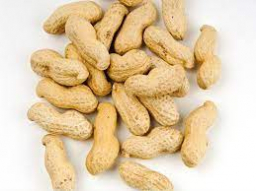Chad buys peanuts in 2-pound bags. He repackages them into bags that hold 5/6 pounds of peanuts. How many 2-pound bags of peanuts should Chad buy to fill the 5/6-pound bags without having any peanuts left over?

x =  5

### Step-by-step explanation:Did you find an error or inaccuracy? Feel free to write us. Thank you!

Tips for related online calculators
Need help calculating sum, simplifying, or multiplying fractions? Try our fraction calculator.
Do you solve Diofant problems and looking for a calculator of Diofant integer equations?
Do you have a linear equation or system of equations and looking for its solution? Or do you have a quadratic equation?